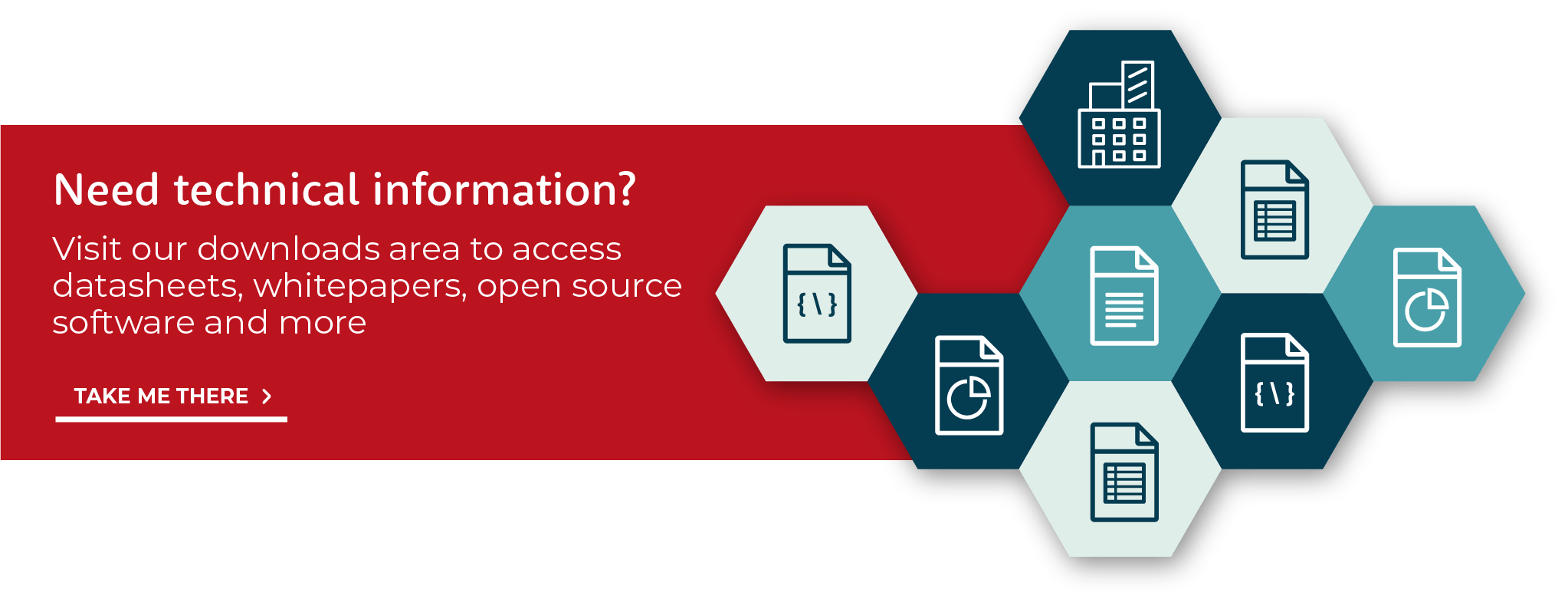Prof. Rob Maunder, the CTO of AccelerComm, discusses how to characterise the performance of channel codes.So in the schematic up here, we can see we have the transmitter, we have the receiver, and we have the wireless channel in between, and the channel can be characterised by a signal to noise ratio. And when we characterise the performance of the channel code, we typically do it in terms of the channel SNR.

Okay, so we have a graph here, we call this a BLER plot, where the x-axis is the signal to noise ratio. So over here represents a strong channel with good channel conditions, over here is a poor channel, and the y-axis for this plot is the block error rate. So this is telling us the fraction of these decoded blocks, here, which will have an error in them. So in the worst case scenario, 100% of these blocks here will have errors, so that's a block error rate of one.

In a more friendly scenario, we might expect a block error rate of 1%, for example, or 10%. So we have a y-axis here, which characterises the error correction performance, and in good channels we expect to have a low block error rate, in poor channels we have a high block error rate.

So we might get a performance that looks something like this. And this might be the performance we have with a short block length, so here the block length is the length of these number of bits we have up here, so when a block length is short, we might get a performance like this, and this might be the performance we get after let's say eight iterations. So the iterations here are performed by the decoder.

The more iterations we perform, the better we can expect the BLER performance to be. So if we instead did 16 iterations, we might get a performance like this, or 32 iterations, we might get that. Okay, so we can have 16, we can have 32. Also the length of the block makes a difference, so if we have a longer block, typically we get a steeper curve, so this might be a long block length.

And so, the performance of the LDPC decoder depends on a lot of things, it depends on the number of iterations, the block length, it also depends on the coding rate, the ratio of A to E. So a high coding rate would be an A which is large compared with E, a low coding rate would be an A which is small compared with E. And so, if we have a low coding rate then we can work in worse channels.

So if we have a low coding rate, we could be over here. So the AccelerComm data briefs characterise the performance of the decoder in terms of block error rate across a wide variety of different scenarios, to make it all crystal clear.
---

We have produced data briefs that characterise the performance of the decoder in terms of block error rate (BLER) across a wide variety of different scenarios.

These can be found within our resources section.